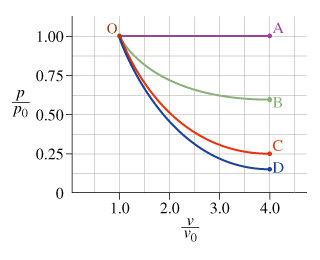# Problem: The following questions refer to the process represented by curve OB, in which an ideal gas proceeds from state O to state B.Part A. The temperature of the system in state B is __________ the temperature of the system in state O.A. greater thanB, less thanC. equal toPart B. The internal energy of the system in state B is __________ the internal energy of the system in state O.A. greater thanB, less thanC. equal to

###### FREE Expert Solution

Part A

Curve OB is between the isobaric and isothermal process.

Since the pressure and the volumes change, the temperature also changes.

From the ideal gas equation, the temperature, T can be approximated by:

T = PV/nR

84% (409 ratings)###### Problem DetailsThe following questions refer to the process represented by curve OB, in which an ideal gas proceeds from state O to state B.

Part A. The temperature of the system in state B is __________ the temperature of the system in state O.

A. greater than
B, less than
C. equal to

Part B. The internal energy of the system in state B is __________ the internal energy of the system in state O.

A. greater than
B, less than
C. equal to